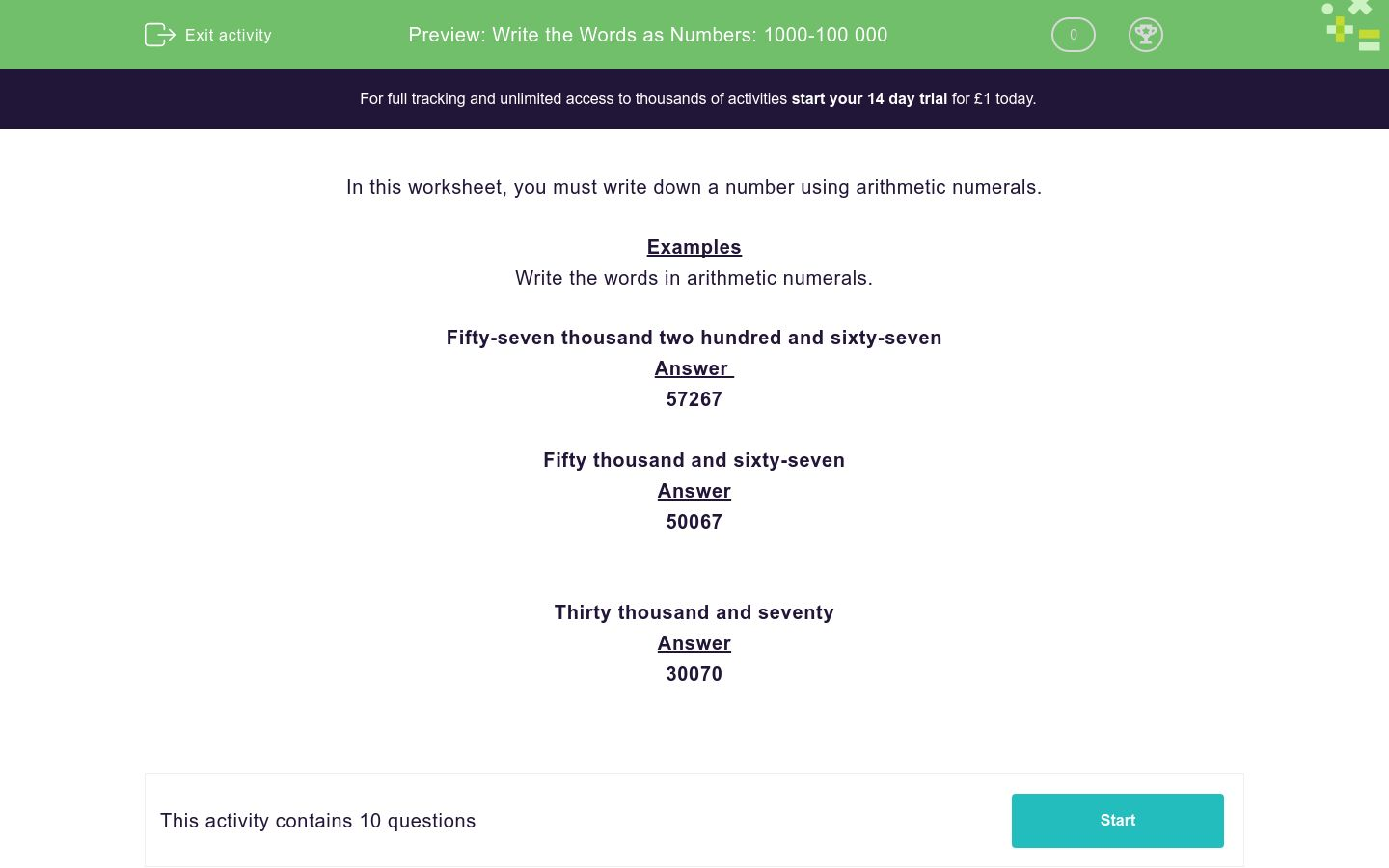# Write the Words as Numbers: 1000-100 000

In this worksheet, students read a long number written in words, then write it again using numbers only.Key stage:  KS 2

Curriculum topic:   Number: Number and Place Value

Curriculum subtopic:   Know Numbers to 1 000 000

Difficulty level:### QUESTION 1 of 10

In this worksheet, you must write down a number using arithmetic numerals.

Examples

Write the words in arithmetic numerals.

Fifty-seven thousand two hundred and sixty-seven

57267

Fifty thousand and sixty-seven

50067

Thirty thousand and seventy

30070

Write the words in arithmetic numerals.

Twenty thousand six hundred and seventy-eight

Write the words in arithmetic numerals.

Twenty-eight thousand one hundred and thirty-eight

Write the words in arithmetic numerals.

Sixty-one thousand four hundred and eighty-three

Write the words in arithmetic numerals.

Eighty-four thousand one hundred and seventy-five

Write the words in arithmetic numerals.

Ninety-six thousand six hundred and forty-nine

Write the words in arithmetic numerals.

Twenty thousand one hundred and sixty-three

Write the words in arithmetic numerals.

Ninety thousand one hundred and five

Write the words in arithmetic numerals.

Seventy-one thousand and ninety-seven

Write the words in arithmetic numerals.

Seventy thousand and seven

Write the words in arithmetic numerals.

Forty thousand and forty

• Question 1

Write the words in arithmetic numerals.

Twenty thousand six hundred and seventy-eight

20678
EDDIE SAYS
Using place value, we know that: 20000 + 600 + 70 + 8 = 20678
• Question 2

Write the words in arithmetic numerals.

Twenty-eight thousand one hundred and thirty-eight

28138
EDDIE SAYS
Using place value, we know that: 20000 + 8000 + 100 + 30 + 8 = 28138
• Question 3

Write the words in arithmetic numerals.

Sixty-one thousand four hundred and eighty-three

61483
EDDIE SAYS
Using place value, we know that: 60000 + 1000 + 400 + 80 + 3 = 61483
• Question 4

Write the words in arithmetic numerals.

Eighty-four thousand one hundred and seventy-five

84175
EDDIE SAYS
Using place value, we know that: 80000 + 4000 + 100 + 70 + 5 = 84175
• Question 5

Write the words in arithmetic numerals.

Ninety-six thousand six hundred and forty-nine

96649
EDDIE SAYS
Using place value, we know that: 90000 + 6000 + 600 + 40 + 9 = 96649
• Question 6

Write the words in arithmetic numerals.

Twenty thousand one hundred and sixty-three

20163
EDDIE SAYS
Using place value, we know that: 20000 + 100 + 60 + 3 = 20163
• Question 7

Write the words in arithmetic numerals.

Ninety thousand one hundred and five

90105
EDDIE SAYS
Using place value, we know that: 90000 + 100 + 5 = 90105
• Question 8

Write the words in arithmetic numerals.

Seventy-one thousand and ninety-seven

71097
EDDIE SAYS
Using place value, we know that: 70000 + 1000 + 90 + 7 = 71097
• Question 9

Write the words in arithmetic numerals.

Seventy thousand and seven

70007
EDDIE SAYS
Using place value, we know that: 70000 + 7 = 70007
• Question 10

Write the words in arithmetic numerals.

Forty thousand and forty

40040
EDDIE SAYS
Using place value, we know that: 40000 + 40 = 40040
---- OR ----

Sign up for a £1 trial so you can track and measure your child's progress on this activity.

### What is EdPlace?

We're your National Curriculum aligned online education content provider helping each child succeed in English, maths and science from year 1 to GCSE. With an EdPlace account you’ll be able to track and measure progress, helping each child achieve their best. We build confidence and attainment by personalising each child’s learning at a level that suits them.

Get started